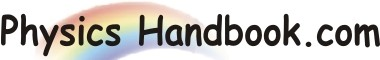HOME TOPICS DEFINITIONS TABLES LAWS INVENTIONS EXPERIMENTS QUIZ VIDEOS
 Hooke's Law Hooke's law of elasticity is an approximation that states that the extension of a spring is in direct proportion with the load added to it as long as this load does not exceed the elastic limit. Hooke's law is named after the British physicist Robert Hooke. For systems that obey Hooke's law, the extension produced is directly proportional to the load, F  =  -k X where, X = The distance that the spring has been stretched or compressed away from the equilibrium position, which is the position where the spring would naturally come to rest (meters). F = The restoring force exerted by the material (newtons) k = The force constant (also termed as spring constant) The unit of force constant is force per unit length (newtons per meter)